# Tutorial: Classification¶

Agenda:

1. Classification running example: Iris Flowers
2. Weight space & feature space intuition
3. Perceptron convergence proof
4. Gradient Descent for Multiclass Logisitc Regression
In :
import matplotlib
import numpy as np
import matplotlib.pyplot as plt
%matplotlib inline


## Classification with Iris¶

We're going to use the Iris dataset.

We will only work with the first 2 flower classes (Setosa and Versicolour), and with just the first two features: length and width of the sepal

If you don't know what the sepal is, see this diagram: https://www.math.umd.edu/~petersd/666/html/iris_with_labels.jpg

In :
from sklearn.datasets import load_iris
print iris['DESCR']

Iris Plants Database

Notes
-----
Data Set Characteristics:
:Number of Instances: 150 (50 in each of three classes)
:Number of Attributes: 4 numeric, predictive attributes and the class
:Attribute Information:
- sepal length in cm
- sepal width in cm
- petal length in cm
- petal width in cm
- class:
- Iris-Setosa
- Iris-Versicolour
- Iris-Virginica
:Summary Statistics:
============== ==== ==== ======= ===== ====================
Min  Max   Mean    SD   Class Correlation
============== ==== ==== ======= ===== ====================
sepal length:   4.3  7.9   5.84   0.83    0.7826
sepal width:    2.0  4.4   3.05   0.43   -0.4194
petal length:   1.0  6.9   3.76   1.76    0.9490  (high!)
petal width:    0.1  2.5   1.20  0.76     0.9565  (high!)
============== ==== ==== ======= ===== ====================
:Missing Attribute Values: None
:Class Distribution: 33.3% for each of 3 classes.
:Creator: R.A. Fisher
:Donor: Michael Marshall (MARSHALL%[email protected])
:Date: July, 1988

This is a copy of UCI ML iris datasets.
http://archive.ics.uci.edu/ml/datasets/Iris

The famous Iris database, first used by Sir R.A Fisher

This is perhaps the best known database to be found in the
pattern recognition literature.  Fisher's paper is a classic in the field and
is referenced frequently to this day.  (See Duda & Hart, for example.)  The
data set contains 3 classes of 50 instances each, where each class refers to a
type of iris plant.  One class is linearly separable from the other 2; the
latter are NOT linearly separable from each other.

References
----------
- Fisher,R.A. "The use of multiple measurements in taxonomic problems"
Annual Eugenics, 7, Part II, 179-188 (1936); also in "Contributions to
Mathematical Statistics" (John Wiley, NY, 1950).
- Duda,R.O., & Hart,P.E. (1973) Pattern Classification and Scene Analysis.
(Q327.D83) John Wiley & Sons.  ISBN 0-471-22361-1.  See page 218.
- Dasarathy, B.V. (1980) "Nosing Around the Neighborhood: A New System
Structure and Classification Rule for Recognition in Partially Exposed
Environments".  IEEE Transactions on Pattern Analysis and Machine
Intelligence, Vol. PAMI-2, No. 1, 67-71.
- Gates, G.W. (1972) "The Reduced Nearest Neighbor Rule".  IEEE Transactions
on Information Theory, May 1972, 431-433.
- See also: 1988 MLC Proceedings, 54-64.  Cheeseman et al"s AUTOCLASS II
conceptual clustering system finds 3 classes in the data.
- Many, many more ...


In :
# code from
# http://stackoverflow.com/questions/21131707/multiple-data-in-scatter-matrix
from pandas.tools.plotting import scatter_matrix
import pandas as pd

iris_data = pd.DataFrame(data=iris['data'],columns=iris['feature_names'])
iris_data["target"] = iris['target']
color_wheel = {1: "#0392cf",
2: "#7bc043",
3: "#ee4035"}
colors = iris_data["target"].map(lambda x: color_wheel.get(x + 1))
ax = scatter_matrix(iris_data, color=colors, alpha=0.6, figsize=(15, 15), diagonal='hist')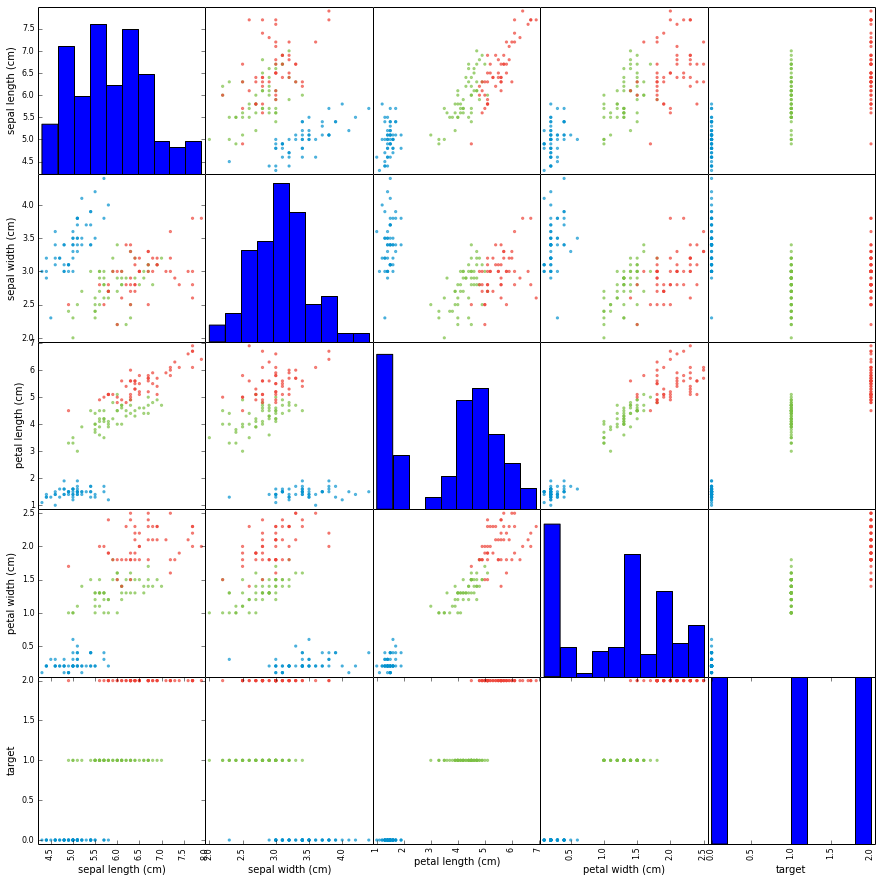In :
# Select first 2 flower classes (~100 rows)
# And first 2 features

sepal_len = iris['data'][:100,0]
sepal_wid = iris['data'][:100,1]
labels = iris['target'][:100]

# We will also center the data
# This is done to make numbers nice, so that we have no
# need for biases in our classification. (You might not
# be able to remove biases this way in general.)

sepal_len -= np.mean(sepal_len)
sepal_wid -= np.mean(sepal_wid)

In :
# Plot Iris

plt.scatter(sepal_len,
sepal_wid,
c=labels,
cmap=plt.cm.Paired)
plt.xlabel("sepal length")
plt.ylabel("sepal width")

Out:
<matplotlib.text.Text at 0x10ec88f50>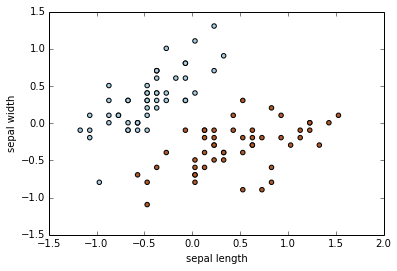### Plotting Decision Boundary¶

Plot decision boundary hypothese

$$w_1 x_1 + w_2 x_2 \ge 0$$

for classification as Setosa.

In :
def plot_sep(w1, w2, color='green'):
'''
Plot decision boundary hypothesis
w1 * sepal_len + w2 * sepal_wid = 0
in input space, highlighting the hyperplane
'''
plt.scatter(sepal_len,
sepal_wid,
c=labels,
cmap=plt.cm.Paired)
plt.title("Separation in Input Space")
plt.ylim([-1.5,1.5])
plt.xlim([-1.5,2])
plt.xlabel("sepal length")
plt.ylabel("sepal width")
if w2 != 0:
m = -w1/w2
t = 1 if w2 > 0 else -1
plt.plot(
[-1.5,2.0],
[-1.5*m, 2.0*m],
'-y',
color=color)
plt.fill_between(
[-1.5, 2.0],
[m*-1.5, m*2.0],
[t*1.5, t*1.5],
alpha=0.2,
color=color)
if w2 == 0: # decision boundary is vertical
t = 1 if w1 > 0 else -1
plt.plot([0, 0],
[-1.5, 2.0],
'-y',
color=color)
plt.fill_between(
[0, 2.0*t],
[-1.5, -2.0],
[1.5, 2],
alpha=0.2,
color=color)

In :
# Example hypothesis
#   sepal_wid >= 0

plot_sep(0, 1)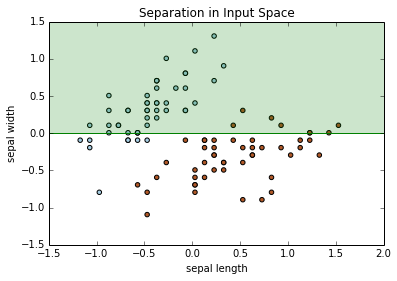In :
# Another example hypothesis:
#   -0.5*sepal_len + 1*sepal_wid >= 0

plot_sep(-0.5, 1)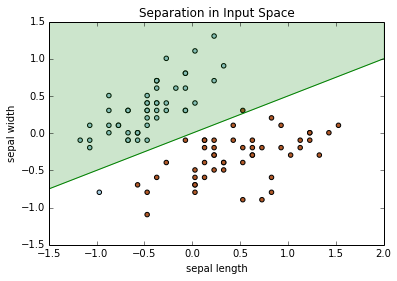In :
# We're going to hand pick one point and
# analyze that point:

a1 = sepal_len
a2 = sepal_wid
print (a1, a2) # (-0.97, -0.79)

plot_sep(-0.5, 1)
plt.plot(a1, a2, 'ob') # highlight the point

(-0.97100000000000097, -0.79400000000000004)

Out:
[<matplotlib.lines.Line2D at 0x10cee6cd0>]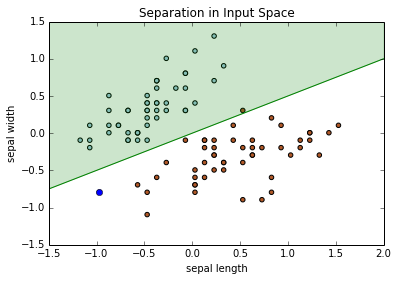### Plot Constraints in Weight Space¶

We'll plot the constraints for some of the points that we chose earlier.

In :
def plot_weight_space(sepal_len, sepal_wid, lab=1,
color='steelblue',
maxlim=2.0):
plt.title("Constraint(s) in Weight Space")
plt.ylim([-maxlim,maxlim])
plt.xlim([-maxlim,maxlim])
plt.xlabel("w1")
plt.ylabel("w2")

if sepal_wid != 0:
m = -sepal_len/sepal_wid
t = 1*lab if sepal_wid > 0 else -1*lab
plt.plot([-maxlim, maxlim],
[-maxlim*m, maxlim*m],
'-y',
color=color)
plt.fill_between(
[-maxlim, maxlim],     # x
[m*-maxlim, m*maxlim], # y-min
[t*maxlim, t*maxlim],      # y-max
alpha=0.2,
color=color)
if sepal_wid == 0: # decision boundary is vertical
t = 1*lab if sepal_len > 0 else -1*lab
plt.plot([0, 0],
[-maxlim, maxlim],
'-y',
color=color)
plt.fill_between(
[0, 2.0*t],
[-maxlim, -maxlim],
[maxlim, maxlim],
alpha=0.2,
color=color)

In :
# Plot the constraint for the point identified earlier:

a1 = sepal_len
a2 = sepal_wid
print (a1, a2)

# Do this on the board first by hand

plot_weight_space(a1, a2, lab=1)

# Below is the hypothesis we plotted earlier
# Notice it falls outside the range.
plt.plot(-0.5, 1, 'og')

(-0.97100000000000097, -0.79400000000000004)

Out:
[<matplotlib.lines.Line2D at 0x10e928fd0>]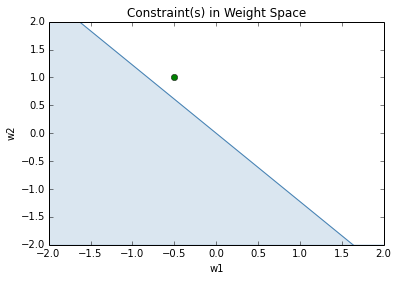### Perceptron Learning Rule Example¶

We'll take one step using the perceptron learning rule

In :
# Using the perceptron learning rule
# TODO: Fill in

w1 = -0.5 # + ...
w2 =  1   # + ...

In :
# This should bring the point closer to the boundary
# In this case, the step brought the point into the
# condition boundary
plot_weight_space(a1, a2, lab=1)
plt.plot(-0.5+a1, 1+a2, 'og')
# old hypothesis
plt.plot(-0.5, 1, 'og')
plt.plot([-0.5, -0.5+a1], [1, 1+a2], '-g')

plt.axes().set_aspect('equal', 'box')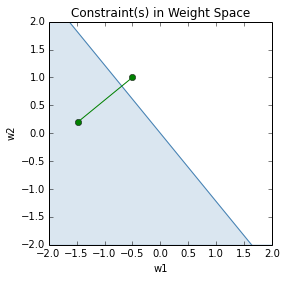In :
# Which means that the point (a1, a2) in input
# space is correctly classified.

plot_sep(-0.5+a1, 1+a2)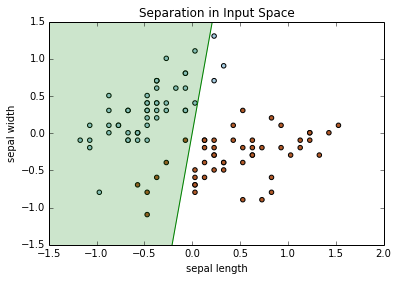### Visualizing Multiple Constraints¶

We'll visualize multiple constraints in weight space.

In :
# Pick a second point
b1 = sepal_len
b2 = sepal_wid

plot_sep(-0.5+a1, 1+a2)
plt.plot(b1, b2, 'or') # plot the circle in red

Out:
[<matplotlib.lines.Line2D at 0x10cc68ed0>]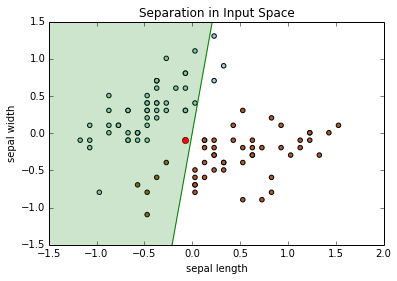In :
# our weights fall outside constraint of second pt.

plot_weight_space(a1, a2, lab=1, color='blue')
plot_weight_space(b1, b2, lab=-1, color='red')
plt.plot(w1, w2, 'ob')

Out:
[<matplotlib.lines.Line2D at 0x10dc8a4d0>]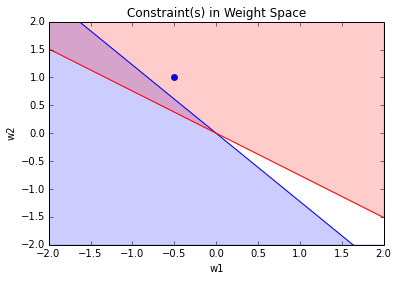In :
# Example of a separating hyperplane
plot_weight_space(a1, a2, lab=1, color='blue')
plot_weight_space(b1, b2, lab=-1, color='red')
plt.plot(-1, 1, 'ok')
plt.show()
plot_sep(-1, 1)
plt.show()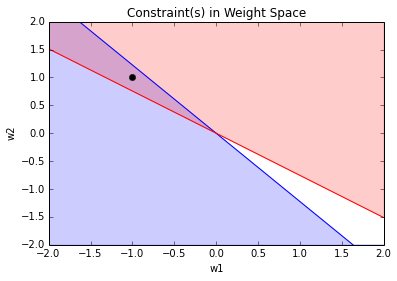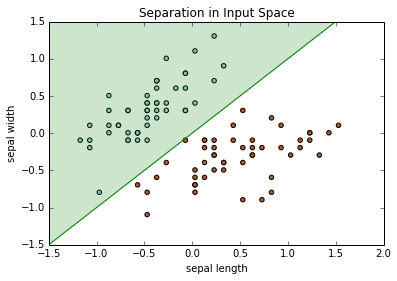## Perceptron Convergence Proof:¶

(From Geoffrey Hinton's slides 2d)

Hopeful claim: Every time the perceptron makes a mistake, the learning algo moves the current weight vector closer to all feasible weight vectors

BUT: weight vector may not get close to feasible vector in the boundary

In :
# The feasible region is inside the intersection of these two regions:
plot_weight_space(a1, a2, lab=1, color='blue')
#plot_weight_space(b1, b2, lab=-1, color='red')

# This is a vector in the feasible region.
plt.plot(-0.3, 0.3, 'ok')

# We started with this point
plt.plot(-0.5, 1, 'og')

# And ended up here
plt.plot(-0.5+a1, 1+a2, 'or')

# Notice that red point is further away to black than the green

plt.axes().set_aspect('equal', 'box')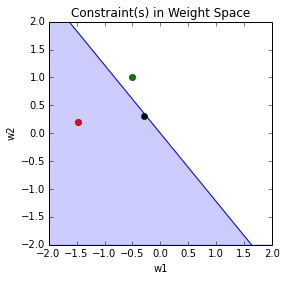• So consider “generously feasible” weight vectors that lie within the feasible region by a margin at least as great as the length of the input vector that defines each constraint plane.
• Every time the perceptron makes a mistake, the squared distance to all of these generously feasible weight vectors is always decreased by at least the squared length of the update vector.
In :
plot_weight_space(a1, a2, lab=1, color='blue' ,maxlim=15)
plot_weight_space(b1, b2, lab=-1, color='red', maxlim=15)

# We started with this point
plt.plot(-0.5, 1, 'og')
plt.plot(-0.5+a1, 1+a2, 'or')
plt.axes().set_aspect('equal', 'box')

# red is closer to "generously feasible" vectors on the top left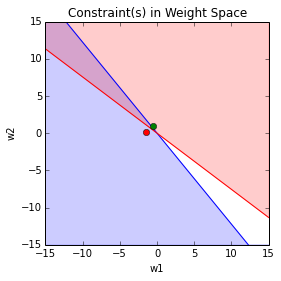### Inform Sketch of Proof of Convergence¶

• Each time the perceptron makes a mistake, the current weight vector moves to decrease its squared distance from every weight vector in the “generously feasible” region.
• The squared distance decreases by at least the squared length of the input vector.
• So after a finite number of mistakes, the weight vector must lie in the feasible region if this region exists.

## Gradient Descent for Multiclass Logisitc Regression¶

Multiclass logistic regression:

\begin{align} {\bf z} &= {\bf W}{\bf x} + {\bf b} \\ {\bf y} &= \text{softmax}({\bf z}) \\ {\mathcal L}_\text{CE} &= -{\bf t}^T(\log \bf{y}) \end{align}

Draw out the shapes on the board before continuing.

In :
# Aside: lots of functions work on vectors

print np.log([1.5,2,3])
print np.exp([1.5,2,3])

[ 0.40546511  0.69314718  1.09861229]
[  4.48168907   7.3890561   20.08553692]


Start by expanding the cross entropy loss so that we can work with it

$${\mathcal L}_\text{CE} = -\sum_l t_l \log(y_l)$$

### Main setup¶

We'll take the derivative with respect to the loss:

\begin{align} \frac{\partial {\mathcal L}_\text{CE}}{\partial w_{kj}} &= \frac{\partial }{\partial w_{kj}} (-\sum_l t_l \log(y_l)) \\ &= -\sum_l \frac{t_l}{y_l} \frac{\partial y_l}{\partial w_{kj}} \end{align}

Normally in calculus we have the rule:

\begin{align} \frac{\partial y_l}{\partial w_{kj}} &= \sum_m \frac{\partial y_l}{\partial z_m} \frac{\partial z_m}{\partial w_{kj}} \end{align}

But $w_{kj}$ is independent of $z_m$ for $m \ne k$, so

\begin{align} \frac{\partial y_l}{\partial w_{kj}} &= \frac{\partial y_l}{\partial z_k} \frac{\partial z_k}{\partial w_{kj}} \end{align}

AND

$$\frac{\partial z_k}{\partial w_{kj}} = x_j$$

Thus

\begin{align} \frac{\partial {\mathcal L}_\text{CE}}{\partial w_{kj}} &= -\sum_l \frac{t_l}{y_l} \frac{\partial y_l}{\partial z_k} \frac{\partial z_k}{\partial w_{kj}} \\ &= -\sum_l \frac{t_l}{y_l} \frac{\partial y_l}{\partial z_k} x_j \\ &= x_j (-\sum_l \frac{t_l}{y_l} \frac{\partial y_l}{\partial z_k}) \\ &= x_j \frac{\partial {\mathcal L}_\text{CE}}{\partial z_k} \end{align}

### Derivative with respect to $z_k$¶

But we can show (on board) that

$$\frac{\partial y_l}{\partial z_k} = y_k (I_{k,l} - y_l)$$

Where $I_{k,l} = 1$ if $k=l$ and $0$ otherwise.

Therefore

\begin{align} \frac{\partial {\mathcal L}_\text{CE}}{\partial z_k} &= -\sum_l \frac{t_l}{y_l} (y_k (I_{k,l} - y_l)) \\ &= -\frac{t_k}{y_k} y_k(1 - y_k) - \sum_{l \ne k} \frac{t_l}{y_l} (-y_k y_l) \\ &= - t_k(1 - y_k) + \sum_{l \ne k} t_l y_k \\ &= -t_k + t_k y_k + \sum_{l \ne k} t_l y_k \\ &= -t_k + \sum_{l} t_l y_k \\ &= -t_k + y_k \sum_{l} t_l \\ &= -t_k + y_k \\ &= y_k - t_k \end{align}

### Putting it all together¶

\begin{align} \frac{\partial {\mathcal L}_\text{CE}}{\partial w_{kj}} &= x_j (y_k - t_k) \end{align}

### Vectorization¶

Outer product.

\begin{align} \frac{\partial {\mathcal L}_\text{CE}}{\partial {\bf W}} &= (\bf{y} - \bf{t}) \bf{x}^T \\ \frac{\partial {\mathcal L}_\text{CE}}{\partial {\bf b}} &= (\bf{y} - \bf{t}) \end{align}
In :
def softmax(x):
#return np.exp(x) / np.sum(np.exp(x))
return np.exp(x - max(x)) / np.sum(np.exp(x - max(x)))

In :
x1 = np.array([1,3,3])
softmax(x1)

Out:
array([ 0.06337894,  0.46831053,  0.46831053])
In :
x2 = np.array([1000,3000,3000])
softmax(x2)

Out:
array([ 0. ,  0.5,  0.5])
In :
def gradient(W, b, x, t):
'''
Gradient update for a single data point.
returns dW and db
This is meant to show how to implement the
obtained equation in code. (not tested)
'''
z = np.matmul(W, x) + b
y = softmax(z)
dW = np.matmul(x, (y-t).T)
db = (y-t)
return dW, db

In [ ]: